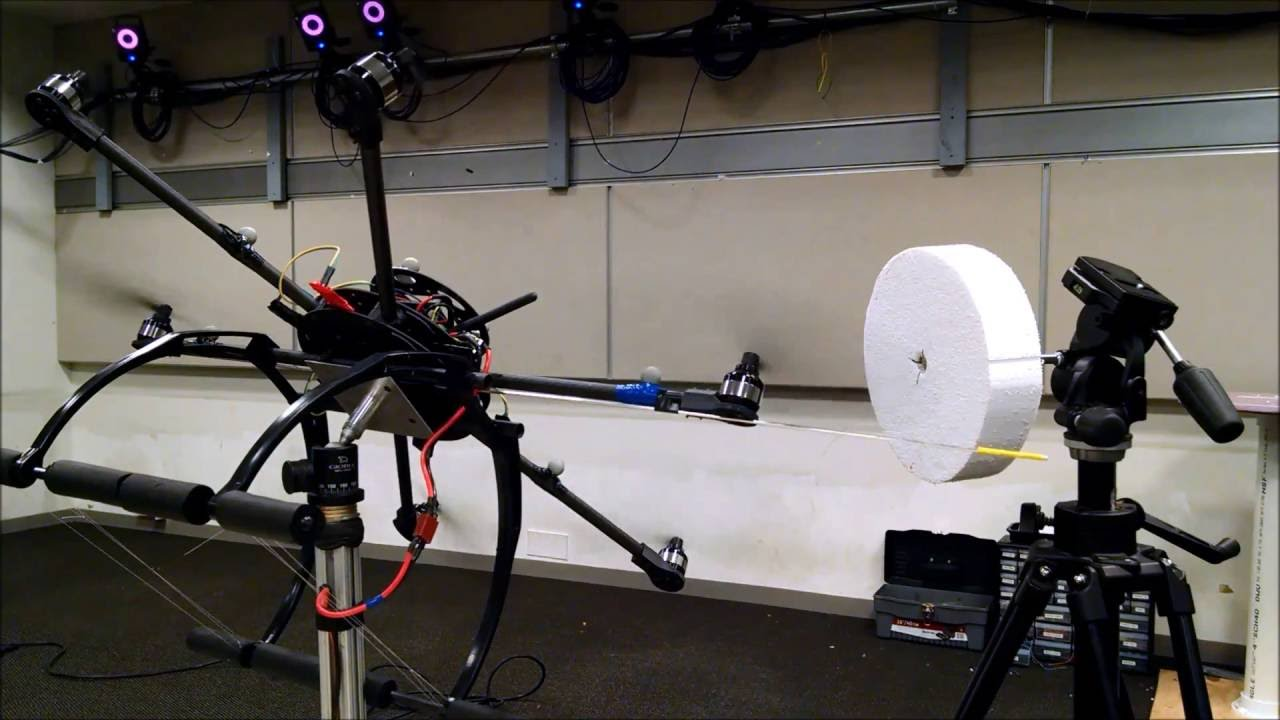# Geometric Adaptive Control of Attitude Dynamics on SO(3) with State Inequality Constraints

$\newcommand{\bracket}{\left[ #1 \right]} \newcommand{\parenth}{\left( #1 \right)} \newcommand{\tr}{\mathrm{tr}\negthickspace\bracket{#1}}$

## Abstract

This paper presents a new geometric adaptive control system with state inequality constraints for the attitude dynamics of a rigid body. The control system is designed such that the desired attitude is asymptotically stabilized, while the controlled attitude trajectory avoids undesired regions defined by an inequality constraint. In addition, we develop an adaptive update law that enables attitude stabilization in the presence of unknown disturbances. The attitude dynamics and the proposed control systems are developed on the special orthogonal group such that singularities and ambiguities of other attitude parameterizations, such as Euler angles and quaternions are completely avoided. The effectiveness of the proposed control system is demonstrated through numerical simulations and experimental results.

## Configuration Error Function

We create a modified configuration error function which utlizes the concept of a barrier function. This error function quantifies the distance between the curent and desired system configuration. The error function, $$\Psi$$ is composed of an attractive term $$A$$ and a repulsive term $$B$$.

\begin{align} \Psi(R, R_d) &= A(R, R_d) B(R) \\ A(R, R_d) &= \frac{1}{2} \tr{G \left( I - R_d^T R\right)} \\ B_i(R) &= 1 - \frac{1}{\alpha_i} \ln \left( - \frac{ r^T R^T v_i - \cos \theta_i}{1 + \cos \theta_i}\right) \end{align}

We can also extend this easily to any arbitrary number of obstacles by slightly modifying our error function:

\begin{align} \Psi = A(R) \bracket{1 + \sum_i C_i(R)} \quad C_i = B(R) - 1 . \end{align}

## Control Design

Using Lyapunov analysis we can design a control input that will ensure that the zero equilibrium is Lyapunov stable. Applying the control input, $$u$$, to the system ensures we can stabilize to the desired attitude while avoiding all obstacles. In addition, we incorporate an adaptive term to handle any uncertainties in the system model.

\begin{align} u &= - k_R e_R - k_{\Omega} e_{\Omega} + \Omega \times J \Omega - W \bar{\Delta}, \\ \dot{\bar{\Delta}} &= k_\Delta W^T \parenth{ e_\Omega + c e_R } . \end{align}
Standard $$\mathsf{SO(3)}$$ controller Constrained $$\mathsf{SO(3)}$$ controllerWe also demonstrate this control system on a robotic vehicle. This video clip shows the hexrotor UAV rotating while avoiding the styrofoam obstacle.@inproceedings{kulumani2016,